Next: 2.2.3 Reemission Up: 2.2 Transport Kinetics Previous: 2.2.1 Reactor-Scale Transport

## 2.2.2 Feature-Scale Transport

Plane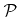together with surfacerepresent the boundary of the feature-scale region (see Figure 2.1). If the arriving flux distribution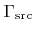is known at, and for all surface points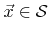the reemitted flux distribution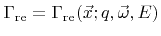is given, the particle transport through the feature-scale region is well-defined. This allows the determination of the arriving angular energy particle flux distribution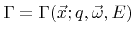on the surface, which principally defines the surface rates. The dependence of the reemitted flux distribution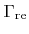on the local arrival flux distribution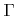will be discussed later.

For most processes the frequency of particle-particle collisions at feature-scale is negligible relative to particle-surface collisions . For comparison, according to (2.4) the mean free path for an ideal gas at room temperature (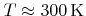) and atmospheric pressure (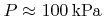) is approximately, which is of the same magnitude as typical sizes of modern structures. However, most dry processes operate at much lower pressures, where the mean free path is much larger than typical feature sizes. This validates the assumption of ballistic transport within the feature-scale region.

The average particle velocities are usually much larger than the surface rates. Kinetic theory gives the mean particle velocity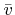of an ideal gas as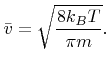(2.13)

For typical temperatures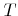and molecular weights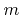the average velocity is larger than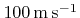. In comparison, the surface rates in most processes are well below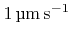. As a consequence, the surface can be regarded to be constant and the travel time of particles can be neglected for calculating the arrival flux distribution at a certain time.

In the ballistic transport regime the particle trajectories of neutral particles are straight lines. For charged particles, such as ions, electromagnetic forces must be incorporated. Incident ions charge insulating layers, such as SiO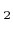. This leads to a static electric field influencing the ion trajectories [30,48]. If the electric field becomes strong enough, the trajectories can be disturbed significantly, which affects the final profile. For example, charging is an essential mechanism for the notching effect which can be observed, if polysilicon-on-insulator structures are overetched . Despite the importance of charging for the description of some effects, this work does not incorporate electromagnetic forces for the intra-feature particle transport. However, as will be outlined in Chapter 7 the main ideas of this work can be extended to incorporate electrostatic fields.

In the absence of electromagnetic forces the trajectories of all particles are straight lines. The particles which arrive at a surface pointwith incident direction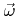can either originate fromor from the surface itself due to reemission, as shown in Figure 2.1. Thus, the particle transport at feature-scale can be described by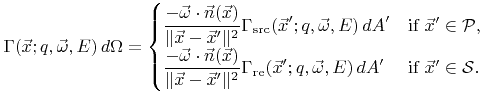(2.14)

Here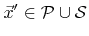is the point seen from point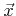in direction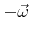, thus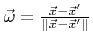.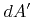is an infinitesimal surface element oforaround.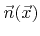is the surface normal at point.Next: 2.2.3 Reemission Up: 2.2 Transport Kinetics Previous: 2.2.1 Reactor-Scale Transport

Otmar Ertl: Numerical Methods for Topography Simulation